# Alternate Notation, Part 1: Converting Hours to Days-Hours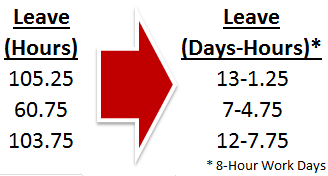Working with numbers is really Excel’s specialty. Most of the basic operations we use involve adding things up, counting them, or performing other basic calculations. This works great when the numbers we use are in the standard base-10 and operate according to the rules of basic arithmetic. Sometimes, though, life gives us a need for strange, inconsistent numbers. Over the course of this series, we’ll explore how to work with Excel in alternative notations, starting today with DaysHours.

## Why Days–Hours Notation?

An hour is mathematically 1/24th of a day, so the proper notation for it in days is 0.041666… But that isn’t very intuitive. Most of the time, when working with dates as quantities, we want to know in terms of our usual notation: days:hours:minutes.  We can use Excel’s date format to do basic arithmetic on times (hours:minutes:seconds), but what if we want to accrue days?

Let’s imagine we’re working with vacation leave numbers. Each employee will have a balance of hours they collect over time, and they’ll “spend” the hours when they take time off. It’s hard to know how much time 66.25 hours really is. What if we want to represent it as the number of full work days?

Normal math would have us divide by 8 hours (one typical work day), and get 8.28125. Great. It’s just over 8 days, but how many hours in remainder?

A better way to show the information we need would be a simple Days-Hours notation. Days would be 8 hours, and Hours would be the remainder.

Here’s are starting worksheet, with 10 employees and their balance of vacation time.## Division Functions: INT and MOD

We’re going to use two Excel functions for basic division to separate out what we need: INT() and MOD(). The syntax for these is simple:

`=INT(number)`
`=MOD(number, divisor)`

INT turns a decimal number into the closest full integer:

`=INT(6.78)`

Rounds down to 6.

`=INT(2.2)`

Rounds down to 2.

`=INT(87)`

Stays 87 because there is no decimal.

MOD tells you what the remainder is after you divide by a number.

`=MOD(43,7)`

Divides 43 by 7, finds that 6 * 7 gets you to 42, and calculates to the remainder of 1.

`=MOD(12,3)`

Divides 12 by 3, finds that 4 * 3 gets you all the way to 12, and calculates to the remainder of 0.

## Converting Hours to Days-Hours

Using our example, we’ll put our Days-Hours notation in column C next to the basic Hours notation in column B. To calculate the number of full days, we’ll divide column B by 8 hours in a business day inside an INT function. This will keep the full days and ignore the confusing partial-day decimal.

`=INT(B2/8)`

To figure the number of remaining hours, we can use the MOD command to do the opposite – ignore the whole number of days and give us the remainder in hours.

`=MOD(B2,8)`

The final cell will stitch the two functions together to achieve the Days-Hours notation we are looking for using the concatenation operator (&):

`=INT(B2/8)&"-"&MOD(B2,8)`

The formula can be dragged down to re-calculate for each employee. The result should look something like the following: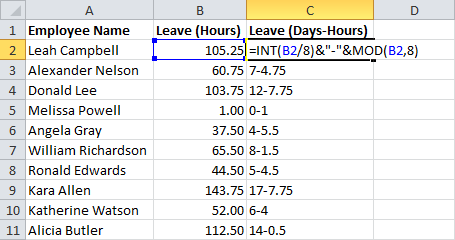## Subtracting in Days–Hours

Done, right? But wait… If we’ve gone through all this trouble to convert our units to DaysHours, we should be able to work with them too… Let’s add a column for planned time off – vacation that employees have already requested that can’t be used for another leave request in the future.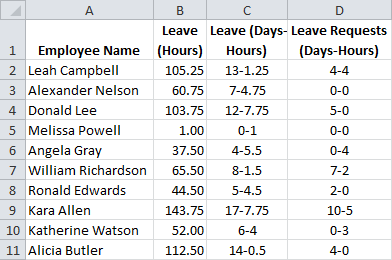Note, if you are adding these manually, Excel may try to change them to dates. You’ll need to set the cell formatting to “Text” for the spreadsheet to leave the columns un-modified.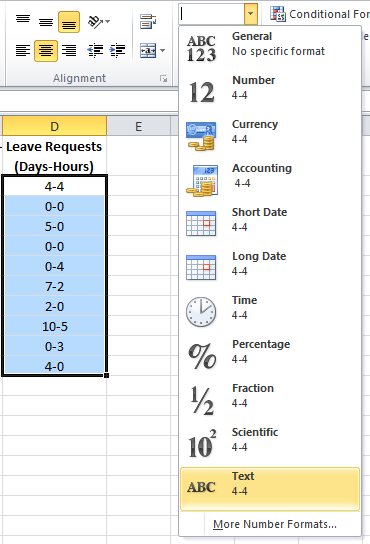## Converting from Days–Hours to Hours Notation

To subtract using our converted notation, we’re going to have to change it back into a form that Excel knows how to do arithmetic with. This means multiplying the days by the number of hours (8), and adding back the remainder. Excel has tools for working with parts of cells, and for this, we’ll use a few: LEFT(), RIGHT(), FIND(), and LEN(). For another example of string parsing, see my article on sorting mixed text.

We can cheat if we want with the first term – we already have the starting leave amounts in hours in column B. But let’s pretend we have to do it the hard way – it’s good to be able to re-use these formulas later.

To isolate the Days term, we need to pull the characters in front of the hyphen (-). LEFT can do this if we know where the hyphen is. For that, we use FIND. The syntax for LEFT and FIND are as follows:

`=FIND(find_text, within_text, [start_num])`
`=LEFT(text, [num_chars])`

Put together, they can pull the number of days from the Days-Hours term:

`=LEFT(C2,FIND("-",C2)-1)`

Multiply by 8 and we have the hours in the Days term. But we still need the remaining hours. We can use RIGHT in the same way for that purpose. The syntax is almost identical to LEFT:

`=RIGHT(text, [num_chars])`

For this, though, we need the difference between the position of the hyphen (from FIND) and the length of the entire string. LEN will do that:

`=LEN(text)`

Using LEN and FIND with RIGHT, we can get the remainder term:

`=RIGHT(C2,LEN(C2)-FIND("-",C2))`

Now we are just left with adding them together:

`=LEFT(C2,FIND("-",C2)-1)*8+RIGHT(C2,LEN(C2)-FIND("-",C2))`

Hurray! All that work and we’re back where we started…

## Subtracting the Converted Terms

But there is some good news. We can let Excel do our work for us to decipher the Leave Requests column we just added.

Just copy the cell we just built (in my example, it’s E2) and paste it in F2. Excel will switch the cell references for you!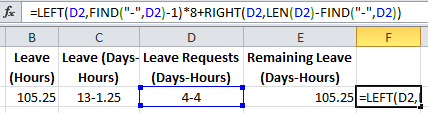This is great, but what we really need to do is subtract the converted Leave Requests (Days-Hours) we built in F2 from the converted Leave (Days-Hours) we built in E2.

We could squeeze all these formulas together into a single cell, and it would work, but why make the formulas more complicated than we need to? We’re already combining 5 functions at a time – let’s make this easier to break down, in case we ever need to look back at it later.

Create a new column after the two conversion columns to do the subtraction (in my example it’s column G). The formula is simple:

=E2F2

Now we’re going to take another shortcut… Remember all that work we did to convert to DaysHours the first time? We’re going to steal it for this calculation. Copy the formula from cell C2. Paste it in H2.

Look at that magic! It converted the Remaining Leave (Hours) to Remaining Leave (Days-Hours)! Now let’s drag-down the formulas to complete the table. Select cells E2:H2, click on the corner, and drag them all down to fill the remaining cells.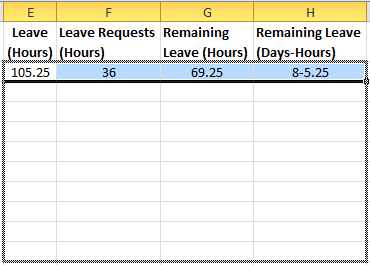Now we did a lot of work to convert from hours to Days-Hours and perform the math on the Leave Requests, but we don’t need to look at it now that it’s done. Select columns E, F, and G. Right click the selection and choose “Hide“.## Employee Leave Days–Hours Example

That’s it! Now your spreadsheet functions completely in Days-Hours! You can look at the data before it was converted on the first worksheet and after on the second spreadsheet. You can also download the sample file by clicking the Excel icon in the bottom right.

## Get the latest Excel tips and tricks by joining the newsletter!Andrew Roberts has been solving business problems with Microsoft Excel for over a decade. Excel Tactics is dedicated to helping you master it.

Join the newsletter to stay on top of the latest articles. Sign up and you'll get a free guide with 10 time-saving keyboard shortcuts!

## 2 thoughts on “Alternate Notation, Part 1: Converting Hours to Days-Hours”

1. Nate says:

How many hours a day would I have to work to equal up to 107hours a week

2. Tom Hanes says:

How can I convert 8975:10 (custom [h]:mm format) into
a years, b months, c days, d hours
and take into account leap years and months of 28-31 days.

With a series of INT and MOD functions strung together, I can do this ASSUMING 30 in every month and 365 days to a year,